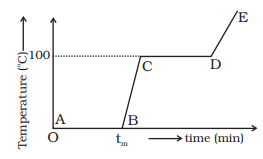# thermal properties of matter important problems

Question 1
the triple points of neon and carbon dioxide are 24.57 k and 216.55K respectively. Express these temperatures on Celsius and Fahrenheit scales

Question 2
Two absolute scales A and B have triple points of water defined as 200A and 350A. What is the relation between TA and TB

Question 3
The pressure of the gas in constant volume gas thermometer are 80 cm, 90 cm and 100 cm of mercury at the ice point, the steam point and in a heated wax bath respectively. Find the temperature of the wax bath.

Question 4
A resistance thermometer reads R= 20.0Ω, 27.5Ω and 50.0 Ω at the ice point (0°C), the steam point (100°C) and the zinc point 420°C respectively. Assuming that the resistance varies with temperature as Rθ=R0(1+αθ+βθ2), find the values of R0, α and β. Here θ represents temperature on Celsius scale.

Question 5
A circular hole of diameter 2.00 cm is made in an aluminium plate at 0°C. What will be the diameter at 100°C. α for aluminium = 2.3 × 10-3 °C-1.

Question 6
The density of water at 0°C is 0.998 gm/cm3 and at 4°C is 1.000 gm/cm3. Calculate the average coefficient of volume expansion of water in temperature range 0 to 4°C.

Question 7
Refer to the below plot of temperature versus time showing the changes in the state of ice on heating (not to scale)Which of the following is correct?
(a) The region AB represents ice and water in thermal equilibrium.
(b) At B water starts boiling.
(c) At C all the water gets converted into steam.
(d) C to D represents water and steam in equilibrium at boiling point.

Question 8
A glass vessel measures exactly 10cm × 10cm × 10cm at 0°C. It is filled completely with mercury at this temperature. When the temperature rises to 10°C , 1.6 cm3 of mercury overflows. Calculate the Coefficient of volume expansion of mercury. Coefficient of linear expansion of glass = 6.5 × 10-6°C-1.

Question 9
A steel rod is clamped at its two ends and rests on a fixed horizontal base. The rod is unstrained at 20°C. Find the longitudinal strain developed in the rod if temperature rises to 50°C. Coefficient of linear expansion of steel is 1.2 × 10-5°C-1

Question 10
To ensure a light fit aluminium reverts used in air-plane construction are made slightly larger than the revert holes and cooled by dry ice (solid CO2) before being driven. If diameter of a hole is 0.2500 in, what should be the diameter of revert at 20°C if is diameter is equal to that of hole when revert is cooled to -78°C the temperature of dry ice? Coefficient of Linear expansion of Aluminium =2.4X10-5°C-1

Question 11
A steel ring of 3.000 in inside diameter at 20°C is to be heated and slipped over a brass shaft measuring 3.002 inch in diameter at 20°C αbrass = 2 × 10-5 °C-1 αsteel =1.2 × 10-5 °C-1
(a) To what temperature should the ring be heated?
(b) If the ring and shaft together are cooled by some means such as liquid air, at what temperature will the ring just slip off the shaft.

Question 12
Specific heat capacity of the a metal A is expressed
as
C=K1T+K2T3
How much heat energy is required to raise the temperature from T0 to 2T0 of unit mass of the metal A
a. (T02/4)(6K1 +15K2T0)
b. (T02/4)(6K1 +15K2T02)
c. (T02/4)(6K1 -15K2T02)
d. None of the above

Question 13
A aluminium container of mass .65 kg contains .35kg of water at 18° C.A block of iron of mass .2kg at 100° C is put into the water. Find the equilibrium temperature of the mixture. Specific heat capacities of aluminium ,iron and water are 910 J/Kg-K,470J/kg-K and 4200 J/kg-K respectively.

Question 14
An iron piece of mass 200 gm is kept inside a furnace for a considerable long time and then put in calorimeter of water equivalent 20 Gm containing 280 gm of water at 22° C. The mixture attains an equilibrium temperature of 80° C. Find the temperature of the furnace.
Specific heat capacities of iron 470 J/kg-° C.

Question 15
1 kg of ice at 0 °C is mixed with 1 kg of steam at 100 °C. What will be the composition of the system when thermal equilibrium is reached. latent heat of fusion of ice = 3.36X105 J/kg and latent heat of vaporization of water = 2.26X106 J/kg.

Question 16
A bullet of mass 30 gm. enters into a fixed wooden block with a speed 50 m / s and stops in it. Calculate the change in internal energy during this process.

Question 17
An animal of 70 kg is running with a speed of 6 m/s. If all the Kinetic energy of animal can be used in increasing water from 18 °C to 32 °C, how much water can be heated with this energy

Question 18
A copper cube of mass 300g slides down on a rough inclined plane of inclination 40 ° at a constant speed. Assume that any loss in Mechanical energy goes into copper block as thermal energy find the increase in thermal energy of the block as it slides down through 50 cm. specific heat capacity of copper = 420J/kg-k. ?